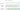# House Robber II

## Problem#

There is a robber planning to rob houses along a street. Each house has some amount of money that can be robbed. All houses at this place are arranged in a circle. That means the first house is the neighbor of the last one. The robber can rob each house except that adjacent houses cannot be robbed since they have security systems connected and it will automatically contact the police.

Given an integer array nums representing the amount of money of each house, return the maximum amount of money the robber can get without alerting the police.

For example,

```Input: nums = [2,3,2]
Output: 3
Explanation: You cannot rob house 1 (money = 2) and then rob house 3 (money = 2), because they are adjacent houses.
```
```Input: nums = [1,2,3,1]
Output: 4
Explanation: Rob house 1 (money = 1) and then rob house 3 (money = 3).
Total amount you can rob = 1 + 3 = 4.
```
```Input: nums = [1,2,3]
Output: 3
```

Constraints:

• `1 <= nums.length <= 100`
• `0 <= nums[i] <= 1000`

## Solution#

This problem is similar to previous House Robber problem. The difference is that the first house is now adjacent to the last one, thus we now have two possibilies of house sequence that can be robbed.Solving this problem is now equal to solve previous House Robber problem with two different ranges:

1. House from `0` to `nums.Length - 2`
2. House from `1` to `nums.Length - 1`

After we get the result from both ranges, then the final result will be the maximum between them.

### Brute Force#

The solution is similar to previous House Robber Brute Force solution where we apply the algorithm to two different ranges:

1. House from `0` to `nums.Length - 2`
2. House from `1` to `nums.Length - 1`

The algorithm works as follows:

1. We compute the `Rob` function for each of the ranges, then we compare the maximum result between them.
2. The `Rob` function will perform recursive call to each of the states.

``````public int Rob(int[] nums)
{
if (nums.Length == 1)
return nums;

if (nums.Length == 2)
return Math.Max(nums, nums);

return Math.Max(Rob(0, nums.Length - 2), Rob(1, nums.Length - 1));

int Rob(int start, int end)
{
if (end - start == 0)
return nums[end];

if (end - start == 1)
return Math.Max(nums[end - 1], nums[end]);

return Math.Max(Rob(start, end - 1), Rob(start, end - 2) + nums[end]);
}
}
``````

#### Complexity Analysis#

Suppose `N` is the length of `nums`.

• Time Complexity: At each step we generate two subtree and the height of the tree is `N`. The total number of generated nodes is 2N. Since, we traverse all the nodes, then the complexity will be O(2N).
• Space Complexity: The complexity will be the same with O(recursion depth), thus it will be O(N).

### Top-down Dynamic Programming#

The solution is also similar to previous House Robber Top Down Dynamic Programming solution where we apply the algorithm to two different ranges:

1. House from `0` to `nums.Length - 2`
2. House from `1` to `nums.Length - 1`

The algorithm works as follows:

1. We compute the `Rob` function for each of the ranges, then we compare the maximum result between them.
2. The `Rob` function will perform memoization so that when the algorithm find repeated states, the result can be retrieved from the memo instead of perform recursive call to recompute the states.

``````public int Rob(int[] nums)
{
if (nums.Length == 1)
return nums;

if (nums.Length == 2)
return Math.Max(nums, nums);

return Math.Max(RobTopDown(nums, 0, nums.Length - 2), RobTopDown(nums, 1, nums.Length - 1));

int RobTopDown(int[] nums, int start, int end)
{
int[] dp = new int;
Array.Fill(dp, -1);

return RobTopDown(start, end);

int RobTopDown(int start, int end)
{
if (end - start == 0)
return nums[end];

if (end - start == 1)
return Math.Max(nums[end - 1], nums[end]);

if (dp[end] > -1)
return dp[end];

int ans = Math.Max(RobTopDown(start, end - 1), RobTopDown(start, end - 2) + nums[end]);
dp[end] = ans;

return ans;
}
}
}
``````

#### Complexity Analysis#

Suppose `N` is the length of `nums`.

• Time Complexity: Since we cache each `Rob(i)`, then we will perform at most N recursive calls, thus the complexity is O(N).
• Space Complexity: The complexity will be the same with O(recursion depth), thus it will be O(N).

### Bottom-up Dynamic Programming#

This approach is the most efficient since we can avoid recursion stack overhead by using `for-loop` iteration linearly.

The solution is also similar to previous House Robber Bottom Up Dynamic Programming solution except that we apply the algorithm to two different ranges:

1. House from `0` to `nums.Length - 2`
2. House from `1` to `nums.Length - 1`

The algorithm works as follows:

1. We compute the `Rob` function for each of the ranges, then we compare the maximum result between them.
2. The `Rob` function will perform iteration linearly where we will perform memoization at ith result in an array. Then the result can be reused to compute future state, i.e.,

`dp[i] = Math.Max(dp[i - 1], dp[i - 2] + nums[i + start])`.

``````public int Rob(int[] nums)
{
if (nums.Length == 1)
return nums;

if (nums.Length == 2)
return Math.Max(nums, nums);

return Math.Max(Rob(0, nums.Length - 2), Rob(1, nums.Length - 1));

int Rob(int start, int end)
{
int[] dp = new int[end - start + 1];

dp = nums[start];
dp = Math.Max(nums[start], nums[start + 1]);

for (int i = 2; i < dp.Length; i++)
{
dp[i] = Math.Max(dp[i - 1], dp[i - 2] + nums[i + start]);
}

return dp[dp.Length - 1];
}
}
``````

#### Complexity Analysis#

Suppose `N` is the length of `nums`.

• Time Complexity: Since we iterate linearly up to `N`, then the complexity is O(N).
• Space Complexity: O(N) since we allocate memory as many as `N` to array `dp` for caching.